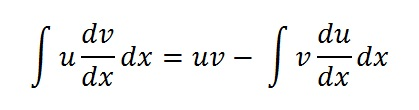# Partial Integration: Definition

The term “partial integration” has several meanings.

1. A synonym for integration by parts (the uv rule), where you substitute two parts of an integral with “u” and “v” to make integration easier :2. A colloquial term for method of partial fractions. The method of partial fractions breaks apart rational functions into a set of simpler rational functions.
3. The inverse of partial differentiation: A method for dealing with integrals in two variable calculus, like iterated integrals. In two variable calculus you have two “partial” derivatives ∂f/∂x (x, y) and ∂f/∂y (x, y), so you have two partial indefinite integrals (integrals are, in a sense, the “opposite of derivatives), hence the term partial integration. The method, also called indefinite integration), works by holding one variable constant. For example, if you have two variables, x and y, you hold one constant (e.g. x) while integrating over y. For an example, see Solving triple integrals.

## Which Definition of Partials Integration Do I Use?

Which definition you use depends on what you’re trying to achieve and which setting you’re in. If you’re in your second semester of calculus, you’ll probably be integrating by parts. If you’re in a multivariable calculus class, the term probably refers to integration of multiple integrals.

That said, there is a lot of ambiguity; There isn’t even a standard definition in textbooks. For example, Markin’s 2008 text Integration For Calculus, Analysis, And Differential Equations  defines partial integration as follows:

Partial integration for definite integral, as well as for indefinite one, consists of applying the formula of integration by parts (p.60).

Larson defines the term quite differently (p.545) :

“For instance, if you are given the partial derivative f(x, y) = 2xy, then, by holding y constant, you can integrate with respect to … called partial integration with respect to x.

If you’re using a textbook, make sure to follow the author’s definition.

## References

 Daileda, R. Integration by Parts. http://ramanujan.math.trinity.edu/rdaileda/teach/s21/m1312/lectures/lecture4_slides.pdf
 Millson, J. Double Integrals. https://www.math.umd.edu/~millson/teaching/STAT400fall18/slides/article17.pdf
 Markin, M. (2018). Integration For Calculus, Analysis, And Differential Equations: Techniques, Examples, And Exercises. World Scientific Publishing Company.
 Larson, R. (2008). Calculus: An Applied Approach. Cengage Learning.

CITE THIS AS:
Stephanie Glen. "Partial Integration: Definition" From StatisticsHowTo.com: Elementary Statistics for the rest of us! https://www.statisticshowto.com/partial-integration/